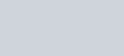Evaluate each of the following integrals as the limit of sums:
Question:

Evaluate each of the following integrals as the limit of sums:

$\int_{0}^{2}(x+4) d x$

Solution: